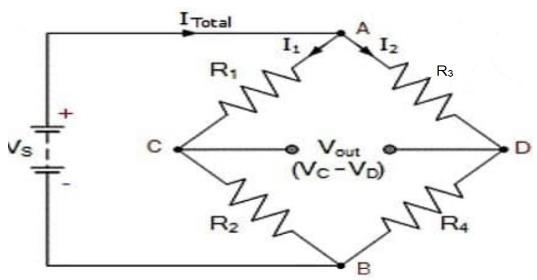Courses

# Test: Kirchhoff’S Rules

## 5 Questions MCQ Test Physics Class 12 | Test: Kirchhoff’S Rules

Description
This mock test of Test: Kirchhoff’S Rules for JEE helps you for every JEE entrance exam. This contains 5 Multiple Choice Questions for JEE Test: Kirchhoff’S Rules (mcq) to study with solutions a complete question bank. The solved questions answers in this Test: Kirchhoff’S Rules quiz give you a good mix of easy questions and tough questions. JEE students definitely take this Test: Kirchhoff’S Rules exercise for a better result in the exam. You can find other Test: Kirchhoff’S Rules extra questions, long questions & short questions for JEE on EduRev as well by searching above.
QUESTION: 1

### At any junction, the sum of the currents entering the junction is equal to the sum of _______

Solution:

Kirchhoff's Current Law or KCL, states that the “total current or charge entering a junction or node is exactly equal to the charge leaving the node as it has no other place to go except to leave, as no charge is lost within the node“. In other words the algebraic sum of ALL the currents entering and leaving a node must be equal to zero, I(exiting) + I(entering) = 0.

QUESTION: 2

### The ______ of changes in potential around any closed loop involving resistors and cells in a loop is zero.

Solution:

In accordance with Kirchhoff’s second law i.e. Kirchhoff’s voltage law (KVL), the algebraic sum of all the potential differences in a closed electric circuit or closed loop that contains one or more cells and resistors is always equal to zero.
This law is popularly called the law of conservation of voltage.

QUESTION: 3

### The Wheatstone bridge Principle is deduced using

Solution:

Kirchoff's Law states that the algebraic sum of currents at a junction of an electric circuit is zero. ... Wheatstone bridge is formed by connecting a battery B or an electric source, a plug key K and a variable resistor X between the junctions A and D and a galvanometer G between the junctions C and D.QUESTION: 4

Given N resistors each of resistance R are first combined to get minimum possible resistance and then combined to get maximum possible resistance. The ratio of the minimum to maximum resistance is​

Solution:

Minimum possible resistance can be found in a parallel arrangement and maximum possible resistance can be found in a series arrangement.
Thus, 1/Rmin=1/R+1/R+....+ n times
=n/R
⇒Rmin=R/n
and Rmax=R+R+.......+ n times
⇒Rmax=nR
∴Rmin/Rmax=(R/n)/nR=1/n2

QUESTION: 5

Two cells of 1.25 V and 0.75V are connected in parallel. The effective voltage will be

Solution:

When two cells are conneted in parallel , then

Effective Voltage,V = V1 − V2 = 1.25 − 0.75 = 0.5 V